## Cauchy Integral Theorem

If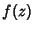is analytic and its partial derivatives are continuous throughout some simply connected region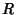, then(1)

for any closed Contourcompletely contained in. Writingas(2)

andas(3)

then gives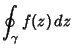(4)

From Green's Theorem,(5)(6)

so (4) becomes(7)

But the Cauchy-Riemann Equations require that(8)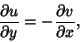(9)

so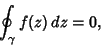(10)

Q. E. D.

For a Multiply Connected region,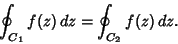(11)

Arfken, G. Cauchy's Integral Theorem.'' §6.3 in Mathematical Methods for Physicists, 3rd ed. Orlando, FL: Academic Press, pp. 365-371, 1985.